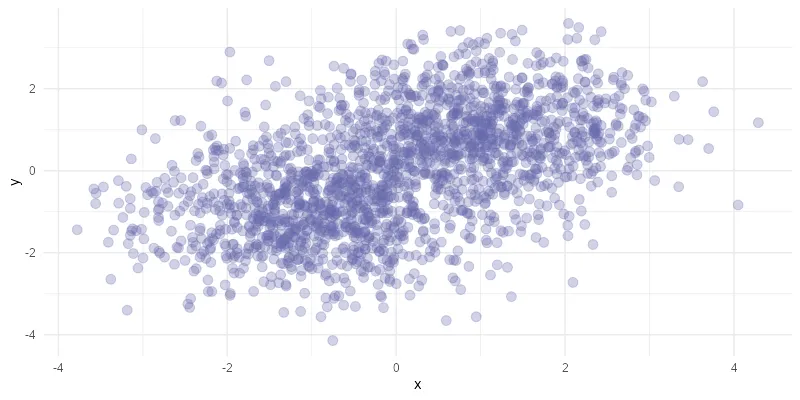# How to save R plots or other results temporarily

If you want to save R plots or other script results temporarily, here is a simple way to do that. It might be helpful if it is necessary to save them outside the R environment, but you don’t want to clean up secondary results. For example, by converting plots to another image format. Here is an example of how to convert images to WebP by using R.

Two functions are helpful, for example, to save R plots temporarily.

With function tempdir, you can see the temporary directory that is created for the current R session.

```tempdir()

# "C:\\Users\\dc\\AppData\\Local\\Temp\\RtmpQbPiA0"```

By the way, this directory contains a subdirectory with files of the plots created in RStudio. You can try to use them if you can locate the necessary ones.

The function tempfile can create a file path for the temporary directory with a keyword in the file name. That is what I will use in this example.

## Save R plot temporarily

Here is an example with an R scatter plot that is created from random data.

```require(ggplot2)

set.seed(12345)

x <- c(rnorm(1000, mean = -1), rnorm(1000, mean = 1))
y <- c(rnorm(1000, mean = -1), rnorm(1000, mean = 1))

xy <- data.frame(x, y)

ggplot(data = xy, aes(x = x, y = y)) +
geom_point(color = "#6667AB",
size = 3,
alpha = 0.3) +
theme_minimal()```If I want to save the R plot temporarily, it is necessary to create a file path with the function tempfile.

```tp_path <- tempfile("myplot_", fileext = ".png")

tp_path

# "C:\\Users\\dc\\AppData\\Local\\Temp\\RtmpQbPiA0\\myplot_43ec63b760a4.png"```

By using this path, you can save the last or necessary plot.

```ggsave(
tp_path,
width = 800,
height = 400,
units = "px",
dpi = 100,
bg = "white"
)```

After that, you can execute other necessary actions. For example, convert to WebP file format. As a result, I can export an R plot with significantly smaller file size and almost the same quality.

```require(webp)
require(png)